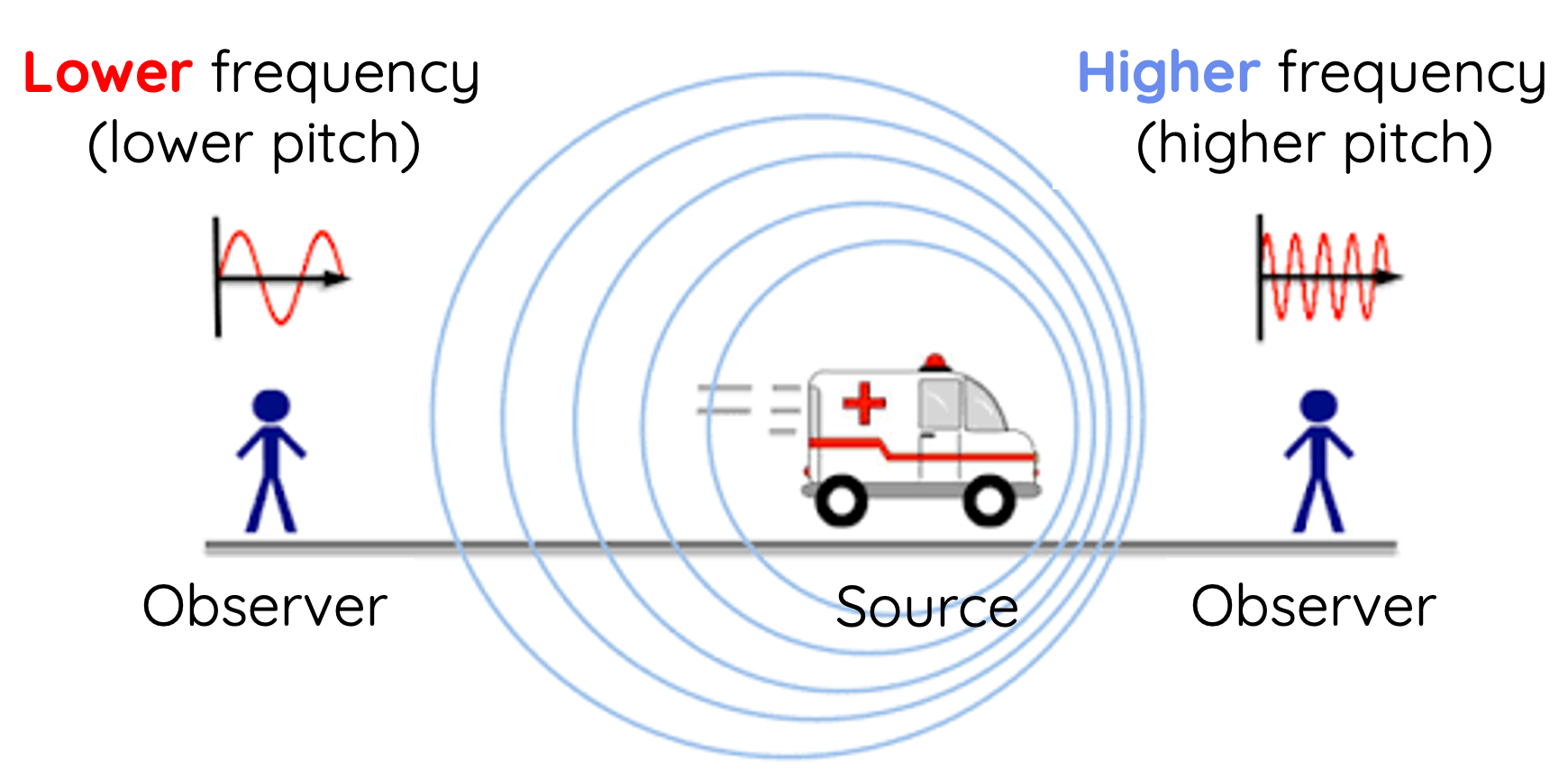# The Doppler Effect

This topic is part of the HSC Physics course under the section Sound Waves.

### HSC Physics Syllabus

• analyse qualitatively and quantitatively the relationships of the wave nature of sound to explain the Doppler's effect

### Understanding the Doppler EffectImagine you're standing on a street corner and an ambulance speeds by with its siren blaring. You've likely noticed that as the ambulance approaches, the siren sounds higher-pitched, but as it passes and moves away, the pitch lowers. This phenomenon is the Doppler Effect at play.

### What is the Doppler Effect?

The Doppler Effect refers to the change in frequency (or wavelength) of a wave concerning an observer who is moving relative to the source of the wave. It's a phenomenon observed with all types of waves, including sound, light, and radio waves.

When the source of a wave is moving towards the observer, the waves get "compressed", leading to a higher frequency (shorter wavelength). Conversely, if the source is moving away from the observer, the waves get "stretched", resulting in a lower frequency (longer wavelength).

For sound waves, a higher frequency is perceived as a higher pitch, while a lower frequency sounds like a lower pitch.

The formula to calculate the observed frequency f' due to the Doppler Effect for any wave including sound is

$$f' = \frac{v_{\text{wave}} + v_{\text{observer}}}{v_{\text{wave}} - v_{\text{source}}} f$$

where

• f = original frequency of the source
• v_{\text{wave}} = velocity of the wave
• v_{\text{observer}} = velocity of the observer relative to the medium (positive if the observer is moving towards the source).
• = velocity of the source relative to the medium (positive if the source is moving towards from the observer).

It is important to be aware that there are various forms of the formula for Doppler's effect which all give the same calculated values when used correctly. HSC Physics students are advised to learn the formula provided above as that is given by the NESA data sheet.

Important values from NESA data sheet:

• The speed of sound in air = 340 m/s
• The speed of light and electromagnetic waves in a vacuum = 3 xx 10^8 m/s

### Calculation Example

An ambulance with a siren frequency of 700 Hz.

if

(a) the ambulance is moving towards a stationary observer at 30 m/s

(b) the ambulance is moving at 30 m/s towards an observer who is moving away from the ambulance at 5 m/s

(c) the ambulance is moving away from a stationary observer at 30 m/s.

Solution to part (a):

$$f' = \frac{v_{\text{wave}} + v_{\text{observer}}}{v_{\text{wave}} - v_{\text{source}}} f$$

Since the ambulance (source) is moving towards the observe, its velocity is positive in the formula:

$$f' = \frac{340 + 0}{340 - 30} \times 700 = 768 \, \text{Hz}$$

Solution to part (b):

Since the observer is moving away from the ambulance, his velocity is negative in the formula:

$$f' = \frac{340 + (-5)}{340 - 30} \times 700 = 756 \, \text{Hz}$$

Solution part (c):

Since the ambulance is moving away from the observer, its velocity is negative in the formula:

$$f' = \frac{340 + 0}{340 - (-30)} \times 700 = 643 \, \text{Hz}$$